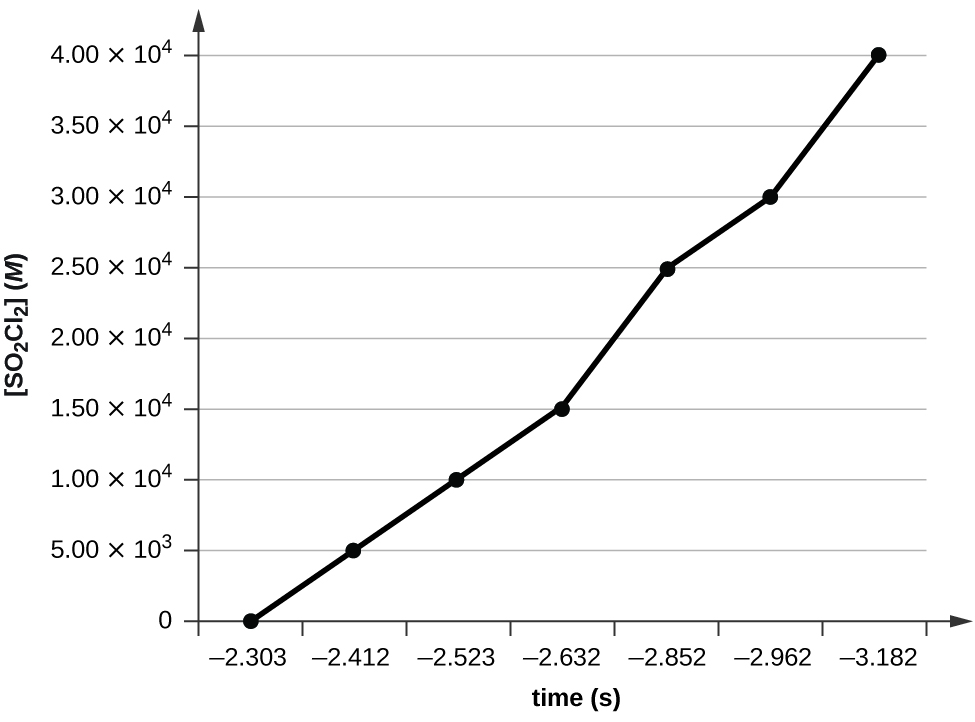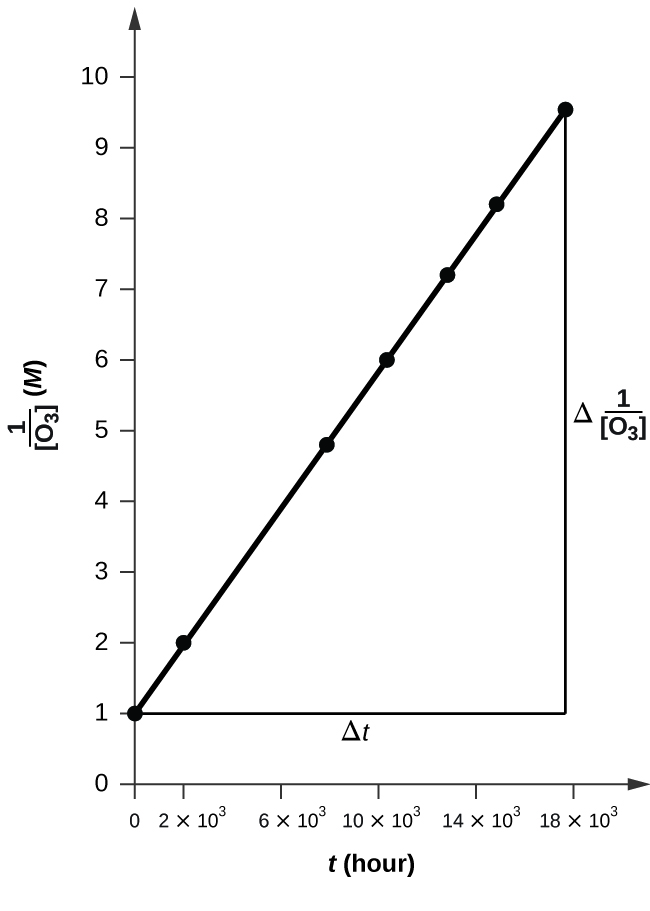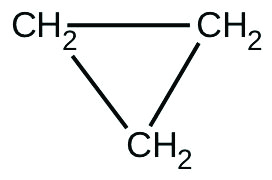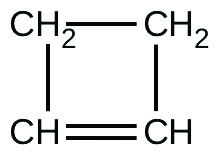# 12.4 Integrated rate laws  (Page 5/7)

 Page 5 / 7

The half-life of a reaction is the time required to decrease the amount of a given reactant by one-half. The half-life of a zero-order reaction decreases as the initial concentration of the reactant in the reaction decreases. The half-life of a first-order reaction is independent of concentration, and the half-life of a second-order reaction decreases as the concentration increases.

## Key equations

• integrated rate law for zero-order reactions: $\left[A\right]=\text{−}kt+{\left[A\right]}_{0},$ ${t}_{1\text{/}2}=\phantom{\rule{0.1em}{0ex}}\frac{{\left[A\right]}_{0}}{2k}$
• integrated rate law for first-order reactions: $\text{ln}\left[A\right]=\text{−}kt+\text{ln}{\left[A\right]}_{0},\phantom{\rule{0.2em}{0ex}}\text{}{t}_{1\text{/}2}=\phantom{\rule{0.1em}{0ex}}\frac{0.693}{k}$
• integrated rate law for second-order reactions: $\frac{1}{\left[A\right]}\phantom{\rule{0.1em}{0ex}}=kt+\phantom{\rule{0.2em}{0ex}}\frac{1}{{\left[A\right]}_{0}},$ ${t}_{1\text{/}2}=\phantom{\rule{0.1em}{0ex}}\frac{1}{{\left[A\right]}_{0}k}$

## Chemistry end of chapter exercises

Describe how graphical methods can be used to determine the order of a reaction and its rate constant from a series of data that includes the concentration of A at varying times.

Use the data provided to graphically determine the order and rate constant of the following reaction: ${\text{SO}}_{2}{\text{Cl}}_{2}\phantom{\rule{0.2em}{0ex}}⟶\phantom{\rule{0.2em}{0ex}}{\phantom{\rule{0.2em}{0ex}}\text{SO}}_{2}+{\text{Cl}}_{2}$

 Time (s) 0 5.00 $×$ 10 3 1.00 $×$ 10 4 1.50 $×$ 10 4 [SO 2 Cl 2 ] ( M ) 0.100 0.0896 0.0802 0.0719 Time (s) 2.50 $×$ 10 4 3.00 $×$ 10 4 4.00 $×$ 10 4 [SO 2 Cl 2 ] ( M ) 0.0577 0.0517 0.0415

Plotting a graph of ln[SO 2 Cl 2 ] versus t reveals a linear trend; therefore we know this is a first-order reaction:k = −2.20 $×$ 10 5 s −1

Use the data provided in a graphical method to determine the order and rate constant of the following reaction:
$2P\phantom{\rule{0.2em}{0ex}}⟶\phantom{\rule{0.2em}{0ex}}Q+W$

 Time (s) 9.0 13.0 18.0 22.0 25.0 [P] ( M ) 1.077 $×$ 10 −3 1.068 $×$ 10 −3 1.055 $×$ 10 −3 1.046 $×$ 10 −3 1.039 $×$ 10 −3

Pure ozone decomposes slowly to oxygen, ${\text{2O}}_{3}\left(g\right)\phantom{\rule{0.2em}{0ex}}⟶\phantom{\rule{0.2em}{0ex}}{\text{3O}}_{2}\left(g\right).$ Use the data provided in a graphical method and determine the order and rate constant of the reaction.

 Time (h) 0 2.0 $×$ 10 3 7.6 $×$ 10 3 1.00 $×$ 10 4 [O 3 ] ( M ) 1.00 $×$ 10 −5 4.98 $×$ 10 −6 2.07 $×$ 10 −6 1.66 $×$ 10 −6 Time (h) 1.23 $×$ 10 4 1.43 $×$ 10 4 1.70 $×$ 10 4 [O 3 ] ( M ) 1.39 $×$ 10 −6 1.22 $×$ 10 −6 1.05 $×$ 10 −6The plot is nicely linear, so the reaction is second order.
k = 50.1 L mol −1 h −1

From the given data, use a graphical method to determine the order and rate constant of the following reaction:
$2X\phantom{\rule{0.2em}{0ex}}⟶\phantom{\rule{0.2em}{0ex}}Y+Z$

 Time (s) 5 10 15 20 25 30 35 40 [ X ] ( M ) 0.099 0.0497 0.0332 0.0249 0.02 0.0166 0.0143 0.0125

What is the half-life for the first-order decay of phosphorus-32? $\left({}_{15}^{32}\text{P}\phantom{\rule{0.2em}{0ex}}⟶\phantom{\rule{0.2em}{0ex}}{}_{16}^{32}\text{S}+{\text{e}}^{-}\right)$ The rate constant for the decay is 4.85 $×$ 10 −2 day −1 .

14.3 d

What is the half-life for the first-order decay of carbon-14? $\left({}_{\phantom{\rule{0.5em}{0ex}}6}^{14}\text{C}⟶{}_{\phantom{\rule{0.5em}{0ex}}7}^{14}\text{N}+{\text{e}}^{-}\right)$ The rate constant for the decay is 1.21 $×$ 10 −4 year −1 .

What is the half-life for the decomposition of NOCl when the concentration of NOCl is 0.15 M ? The rate constant for this second-order reaction is 8.0 $×$ 10 −8 L/mol/s.

8.3 $×$ 10 7 s

What is the half-life for the decomposition of O 3 when the concentration of O 3 is 2.35 $×$ 10 −6 M ? The rate constant for this second-order reaction is 50.4 L/mol/h.

The reaction of compound   A to give compounds   C and   D was found to be second-order in   A . The rate constant for the reaction was determined to be 2.42 L/mol/s. If the initial concentration is 0.500 mol/L, what is the value of t 1/2 ?

0.826 s

The half-life of a reaction of compound A to give compounds D and E is 8.50 min when the initial concentration of A is 0.150 mol/L. How long will it take for the concentration to drop to 0.0300 mol/L if the reaction is (a) first order with respect to A or (b) second order with respect to A ?

Some bacteria are resistant to the antibiotic penicillin because they produce penicillinase, an enzyme with a molecular weight of 3 $×$ 10 4 g/mol that converts penicillin into inactive molecules. Although the kinetics of enzyme-catalyzed reactions can be complex, at low concentrations this reaction can be described by a rate equation that is first order in the catalyst (penicillinase) and that also involves the concentration of penicillin. From the following data: 1.0 L of a solution containing 0.15 µg (0.15 $×$ 10 −6 g) of penicillinase, determine the order of the reaction with respect to penicillin and the value of the rate constant.

[Penicillin] ( M ) Rate (mol/L/min)
2.0 $×$ 10 −6 1.0 $×$ 10 −10
3.0 $×$ 10 −6 1.5 $×$ 10 −10
4.0 $×$ 10 −6 2.0 $×$ 10 −10

The reaction is first order.
k = 1.0 $×$ 10 7 mol −1 min −1

Both technetium-99 and thallium-201 are used to image heart muscle in patients with suspected heart problems. The half-lives are 6 h and 73 h, respectively. What percent of the radioactivity would remain for each of the isotopes after 2 days (48 h)?

There are two molecules with the formula C 3 H 6 . Propene, ${\text{CH}}_{3}\text{CH}={\text{CH}}_{2},$ is the monomer of the polymer polypropylene, which is used for indoor-outdoor carpets. Cyclopropane is used as an anesthetic:When heated to 499 °C, cyclopropane rearranges (isomerizes) and forms propene with a rate constant of
5.95 $×$ 10 −4 s −1 . What is the half-life of this reaction? What fraction of the cyclopropane remains after 0.75 h at 499.5 °C?

4.98; 20% remains

Fluorine-18 is a radioactive isotope that decays by positron emission to form oxygen-18 with a half-life of 109.7 min. (A positron is a particle with the mass of an electron and a single unit of positive charge; the equation is ${}_{518}^{\phantom{\rule{1em}{0ex}}9}\text{F}⟶{}_{18}^{\phantom{\rule{0.5em}{0ex}}8}\text{O}+{\text{e}}^{-}.\right)$ Physicians use 18 F to study the brain by injecting a quantity of fluoro-substituted glucose into the blood of a patient. The glucose accumulates in the regions where the brain is active and needs nourishment.

(a) What is the rate constant for the decomposition of fluorine-18?

(b) If a sample of glucose containing radioactive fluorine-18 is injected into the blood, what percent of the radioactivity will remain after 5.59 h?

(c) How long does it take for 99.99% of the 18 F to decay?

Suppose that the half-life of steroids taken by an athlete is 42 days. Assuming that the steroids biodegrade by a first-order process, how long would it take for $\frac{1}{64}$ of the initial dose to remain in the athlete’s body?

252 days

Recently, the skeleton of King Richard III was found under a parking lot in England. If tissue samples from the skeleton contain about 93.79% of the carbon-14 expected in living tissue, what year did King Richard III die? The half-life for carbon-14 is 5730 years.

Nitroglycerine is an extremely sensitive explosive. In a series of carefully controlled experiments, samples of the explosive were heated to 160 °C and their first-order decomposition studied. Determine the average rate constants for each experiment using the following data:

 Initial [C 3 H 5 N 3 O 9 ] ( M ) 4.88 3.52 2.29 1.81 5.33 4.05 2.95 1.72 t (s) 300 300 300 300 180 180 180 180 % Decomposed 52 52.9 53.2 53.9 34.6 35.9 36 35.4

[ A ] 0 ( M ) k $×$ 10 3 (s −1 )
4.88 2.45
3.52 2.51
2.29 2.54
1.81 2.58
5.33 2.35
4.05 2.44
2.95 2.47
1.72 2.43

For the past 10 years, the unsaturated hydrocarbon 1,3-butadiene $\left({\text{CH}}_{\text{2}}=\text{CH}–\text{CH}={\text{CH}}_{2}\right)$ has ranked 38th among the top 50 industrial chemicals. It is used primarily for the manufacture of synthetic rubber. An isomer exists also as cyclobutene:The isomerization of cyclobutene to butadiene is first-order and the rate constant has been measured as 2.0 $×$ 10 −4 s −1 at 150 °C in a 0.53-L flask. Determine the partial pressure of cyclobutene and its concentration after 30.0 minutes if an isomerization reaction is carried out at 150 °C with an initial pressure of 55 torr.

What is a mole?
pls give me 3 type of transition metal
Copper, Scandium, Vanadium, Iron, Chromium, Cobalt
Jumaane-Kalif
ion zinc hydrogen
Abdul
that is not true
Jumaane-Kalif
stop posting foolishness
Jumaane-Kalif
how do I name compounds
depends on the compound. as you may know there's transition metal compounds and there's organic compounds and so on and so forth.
Jumaane-Kalif
what is electricity
electricity refers to the flow electrons
John
Hi. please can you tell me more on chemical equation
When 1 or 2 chemicals react, they rearrange their atomic composition forming new compounds. The total mass before and after is the same/ constant. Chemical equations of same reactants react in same ratios e.g. 1 Na ion reacts with 1 water molecule or a multipier like 1 mole of Na ions react with 1
Abdelkarim
Mole of water molecules. In that example we multipied by 6.02*10^23 or avogadro constant (L). Or 2 Na+ ions with two water molecules. The arrow means '' to form '' Some times conditions or reactants are written above the arrow like H+ or enzyme or temper, sometimes physical states are written
Abdelkarim
Beside the chemical Aqueous (aq) which means solute dissolved on water. Solid (s) etc Some chemical equations are written next to it ΔH= # which means enthalpy change which describes if the reaction is endothermic (+) or exothermic (-).
Abdelkarim
Some are reversible and have half double arrow sign.
Abdelkarim
what is the meaning of atoma
what is theory
what is chemical compound
Lorrita
A compound is the result of chemical bonding between 2 or more different elements.
Abdelkarim
difference between Amine and amide
what is the difference between alkanal and alkanone
difference between alkanol and alkanal
Michael
whaatt
Saturday
you are not well at all
Ibrahim
is organic compounds used for drying agent
Sulfuric acid is used as a drying agent.
Abdelkarim
what is an atom
An atom is the smallest part of an element, for instance gold atoms are the smallest part of gold that can enter a reaction. An atom must consist protons and electrons of equal number. You can think of those subatomic particles as spheres, but not orbiting randomly they move in specific way in
Abdelkarim
That was partially described mathematically. As a muslim, we believe that god created all matter from nothing. He is the Able, and only who can create matter in the begging in the big bang that was described in the Quran in chapter 17 verse 30, 1400 years ago, you can read it from internet.
Abdelkarim
what is electron affinity
John
do you have a private jet
Ibrahim
what is acid
Proton donor (H+). Like in lemons, oranges and some medicines.
Abdelkarim
what is titration? and how can i get my readings?
what is electrolysis?
what is the formula you use when calculating for gas law?
favor
PV=nrt
Farid
electro-means electricity while lysis-means splitting up so electrolysis simply means splitting up by means of electric current
John
good day. how may I see previous question asked in this chat, please?
Asha
Asha, A person named Favor asked what is meant by the term electrolysis
John
what is a sample
According to google, a speciemen taken for specific testing or analysis.
Abdelkarim

#### Get Jobilize Job Search Mobile App in your pocket Now!ByByBy OpenStaxBy Yacoub JayoghliBy JavaChamp TeamBy Vanessa SoledadBy Frank LevyBy OpenStaxBy Mackenzie WilcoxBy Sam LuongBy Jugnu KhanBy John Gabrieli